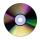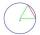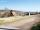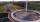Cable

Cable consists of 8 strands, each strand consists of 12 wires with diameter d = 0.5 mm. Calculate the cross-section of the cable.

Result

S =  18.8 mm2

Solution:Leave us a comment of example and its solution (i.e. if it is still somewhat unclear...):Be the first to comment!Next similar examples:

1. LawnsBefore a sports hall are two equally large rectangular lawns, each measuring 40 m and 12 m. Maintenance of 10m² lawn cost 45 CZK yearly. On each lawn is circular flowerbed with a diameter 8 meters. How much money is needed each year to take on lawn care?.
2. SidewalkThe city park is a circular bed of flowers with a diameter of 8 meters, around it the whole length is 1 meter wide sidewalk . What is the sidewalk area?
3. 22/7 circleCalculate approximately area of a circle with radius 20 cm. When calculating π use 22/7.
4. Area of ditchHow great content area will have a section of trapezoidal ditch with a width of 1.6 meters above and below 0.57 meters? The depth of the ditch is 2.08 meters.
5. Pipe cross sectionThe pipe has an outside diameter 1100 mm and the pipe wall is 100 mm thick. Calculate the cross section of this pipe.
6. Circle r,DCalculate the diameter and radius of the circle if it has length 52.45 cm.
7. CD discA compact disc has a diameter of 11.8 cm. What is the surface area of the disc in square centimeters?
8. Diameter to areaFind the area of a circle whose diameter is 26cm.
9. ChordPoint on the circle is the end point of diameter and end point of chord length of radius. What angle between chord and diameter?
10. AnnulusThe radius of the larger circle is 8cm, the radius of smaller is 5cm. Calculate the contents of the annulus.
11. AcreagePlot has a diamond shape, its side is 25.6 m long and the distance of the opposite sides is 22.2 meters. Calculate its acreage.
12. Circle - easy 2The circle has a radius 6 cm. Calculate:
13. Circle - simpleCalculate the area of a circle in dm2, if its circumference is 31.4 cm.
14. AnnulusCalculate the area of two circles annulus k1 (S, 3 cm) and k2 (S, 5 cm).
15. CircleWhat is the radius of the circle whose perimeter is 6 cm?
16. ClassIn 7.C clss are 10 girls and 20 boys. Yesterday was missing 20% of girls and 50% boys. What percentage of students missing?Combine like terms 4c+c-7c